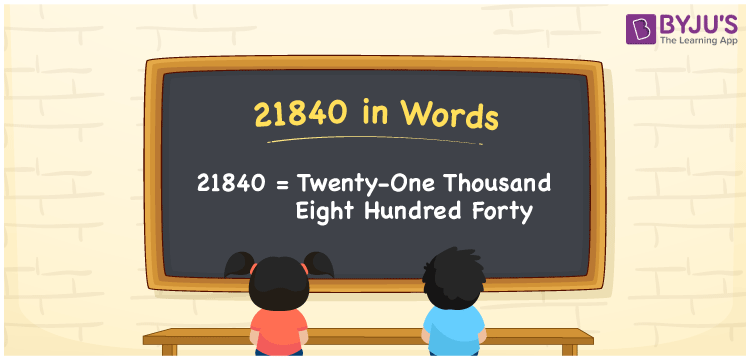# 21840 in Words

We can write 21840 in words as Twenty-one thousand eight hundred forty. Thus, Twenty-one thousand eight hundred forty is the word form of 21840. Also, we know that the word form of a number is simply the number name and is used to spell or read the given number. For example, Rs. 21840 is spelt as Twenty-one thousand eight hundred forty rupees. Let’s understand how to derive the word form of 21840 here in this article.

 21840 in words Twenty-one thousand eight hundred forty Twenty-one thousand eight hundred forty in Numbers 21840

## 21840 in English words

Generally, we write numbers in words using the English alphabet. Thus, we spell 21840 in English as “Twenty-one thousand eight hundred forty”.## How to Write 21840 in Words?

To convert the number 21840 to word form, we must create a place value chart with five columns since it is a five-digit number.

 Ten thousands Thousands Hundreds Tens Ones 2 1 8 4 0

Here, ones = 0, tens = 4, hundreds = 8, thousands = 1, ten thousands = 2

By expanding the above digits, we get;

2 × Ten thousand + 1 × Thousand + 8 × Hundred + 4 × Ten + 0 × One

= 2 × 10000 + 1 × 1000 + 8 × 100 + 4 × 10 + 0 × 1

= 20000 + 1000 + 800 + 40

= Twenty thousand + One thousand + Eight hundred + Forty

= Twenty-one thousand eight hundred forty

Therefore, 21840 in words = Twenty-one thousand eight hundred forty.

As we know, 21840 is a natural number that is the successor of 21839 and the predecessor of 21841.

21840 in words – Twenty-one thousand eight hundred forty

Is 21840 an odd number? – No

Is 21840 an even number? – Yes

Is 21840 a perfect square number? – No

Is 21840 a perfect cube number? – No

Is 21840 a prime number? – No

Is 21840 a composite number? – Yes

## Frequently Asked Questions on 21840 in Words

Q1

### How do you write 21840 in words?

We can write the number 21840 in words as Twenty-one thousand eight hundred forty.
Q2

### How to write an amount of Rs. 21840 in words?

We can write an amount of Rs. 21840 in words as Twenty-one thousand eight hundred forty rupees.
Q3

### What is the word form of 21840?

The word form of the number 21840 is Twenty-one thousand eight hundred forty.